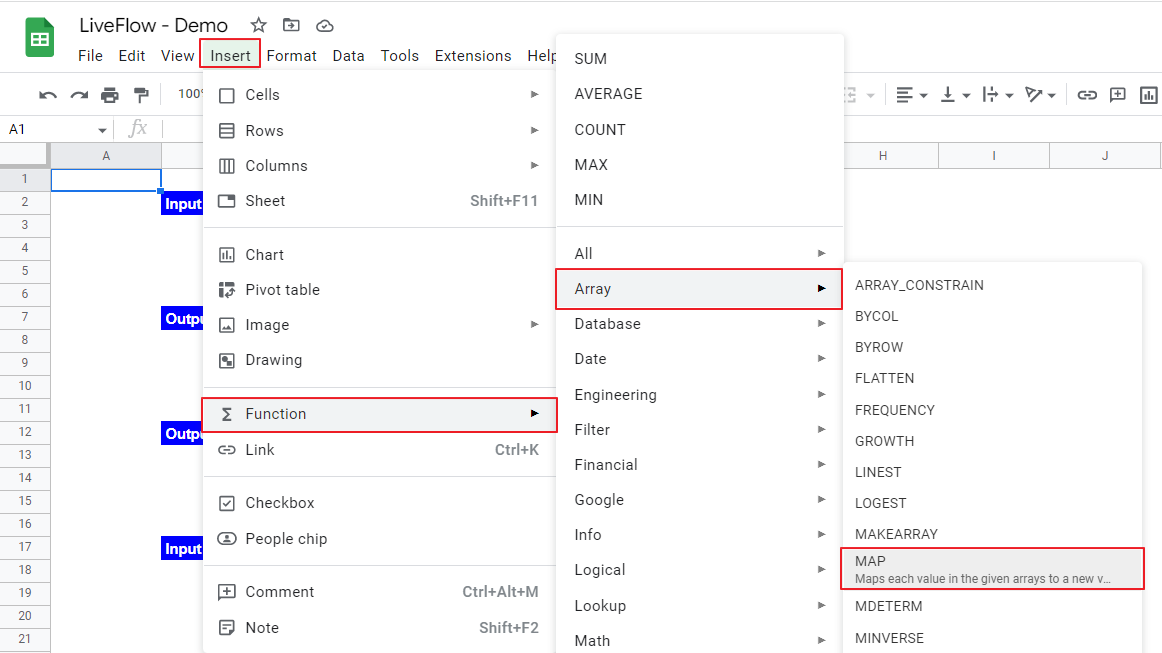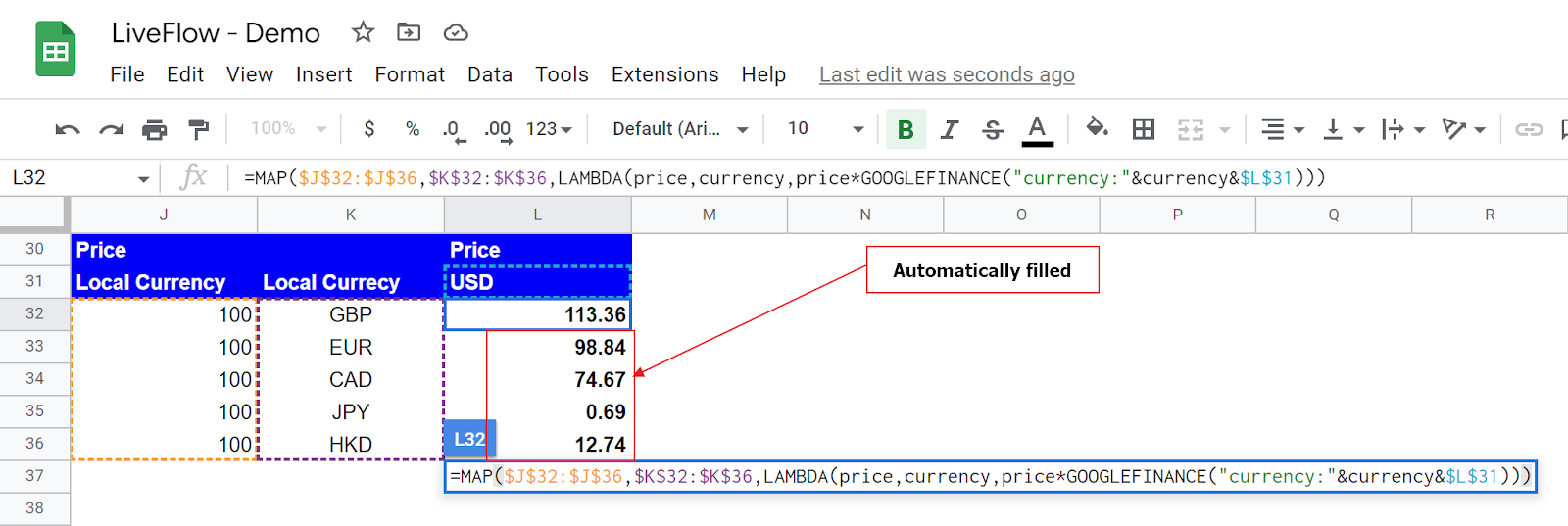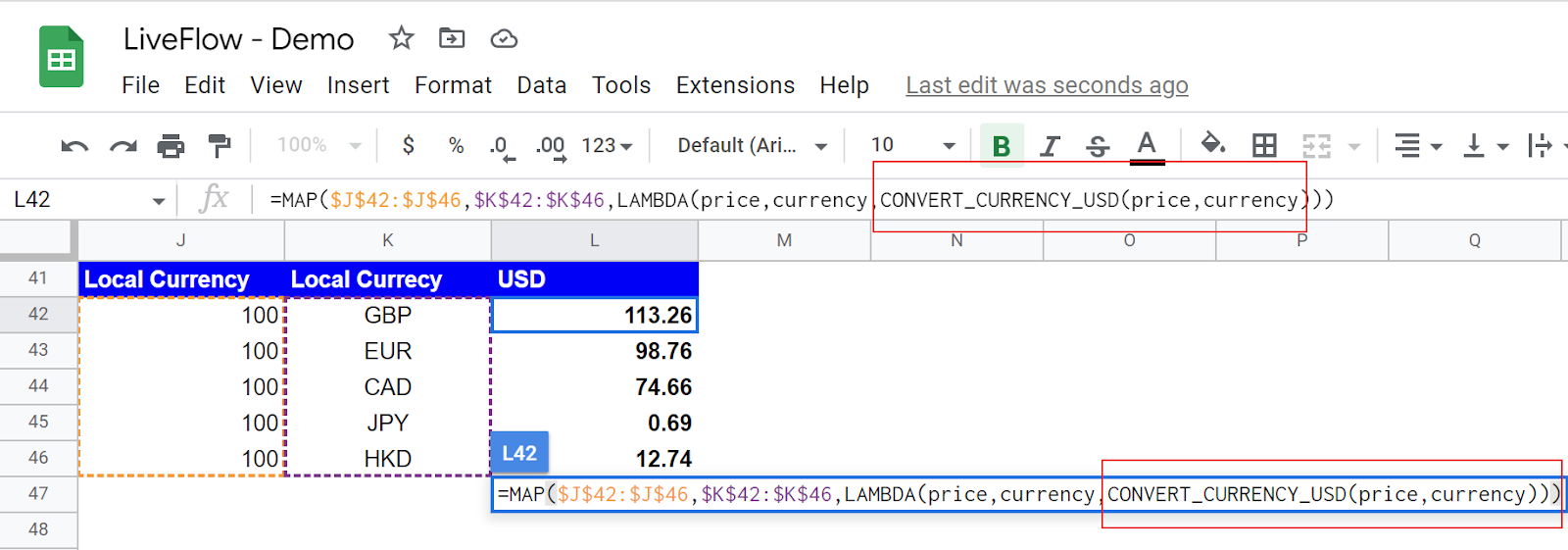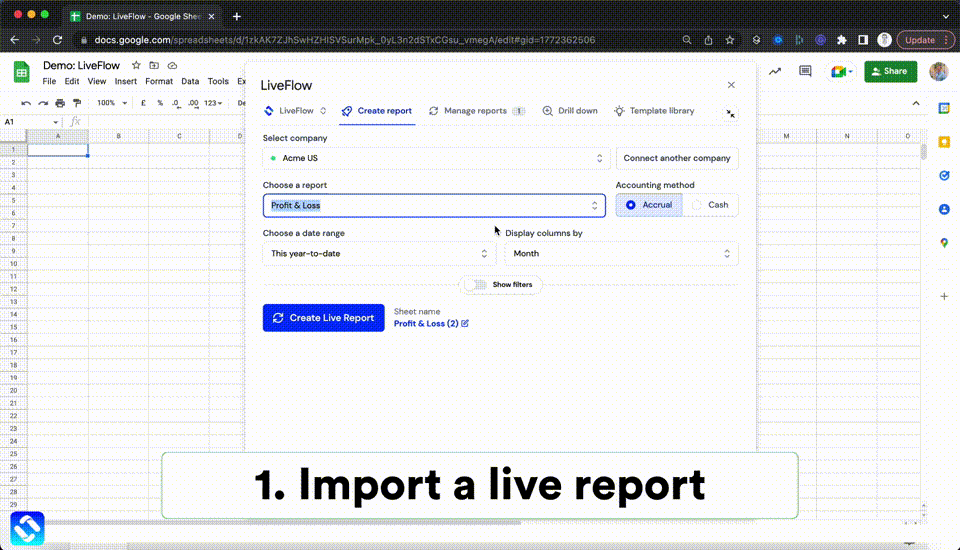# How to Use MAP Function in Google Sheets

In this article, you will learn the MAP function and how to use it in Google Sheets. This function is meant to be used with the LAMBDA function. The MAP formula runs the LAMBDA function for each value in a selected range and returns each result in the same dimensional field (but in different cells).

## How to insert the MAP formula in Google Sheets

1. Type “=MAP” or go to “Insert”“Function”“Array”“MAP”.
2. Choose a range that includes input values.
3. Enter a LAMBDA function with placeholders and logic.
4. Press the “Enter” key.How to insert the MAP function in Google Sheets

## What is the MAP formula in Google Sheets?

The MAP function is beneficial when you want to process values in a range by getting them through formulas and get the processed results automatically in the same size of the dimensional area as that of the one where the original input values are.

The generic syntax is as follows:

``=MAP(array1, [array2, …], lambda)``

Array 1~: This is a range where the LAMBDA function defined in “lambda” operates. If you enter more than one array, note that you need to incorporate the corresponding number of placeholders in the LAMBDA function you will insert. Also, when you include multiple ranges, their sizes should be equal.

Lambda: Input the LAMBDA function such as “LAMBDA(x, x^2)”. You can also use your Named Functions here.

Assume you have a list of values in local currencies, want to convert them to USD, and show them in the same table.How to use the MAP function in Google Sheets

Array 1: J32:J36, this range corresponds to the “price” parameter in the LAMBDA function

Array 2: K32:K36, this range corresponds to the “currency” parameter in the LAMBDA function

Lambda: LAMBDA(price, currency, price*GOOGLEFINANCE(“currency:”&currency&\$L\$31))

*Note: “currency:” is not a parameter but a required text for the GOOGLEFINANCE formula.

Once you enter the MAP formula in L32, you automatically get the returns in L32:L36.

If you use the Named Function in “lambda” section, the entire MAP function looks much simpler. Go to this article to learn how to use the Named Function.How to use the MAP function with Named Functions in Google Sheets

If you want to know more about how this function works in Google Sheets, move on to the next section below.

## How MAP function works in Google Sheets

Move on to the further examples in this section to understand how the MAP function works better.

Input A

This is a 3x3 array containing nine numbers, which will be input for Output A-1 and A-2.

Output A -1

The LAMBDA function in this sample returns InputA^2 for each input. Assume you want to show the calculation results in a range of B8:D10 with the input of each value in the “Input A” array. (e.g., Cell C8 shows the calculation results with the input of 2 in cell C3). As this sample is an intermediate step to explaining how the MAP function works with the LAMBDA function, we applied the LAMBDA function to each cell in the selected range (B8:D10).

The point is you can get the same results with one MAP function.

Output A -2

This table shows what the returns of the MAP function look like. We only inserted one MAP function in cell B13, but it automatically returns nine values in cells in the array of B13:D15, each of which comes from the original input value in the same dimensional position in Input A.

We understand that if a calculation is simple like this example, you think a more straightforward formula without the LAMBDA or MAP function is much easier and quicker. However, we believe these examples are easy enough to learn how the MAP function works with the LAMBDA formula.\

## Other LAMBDA support functions

Check the articles below to learn about other LAMBDA helper functions such as MAKEARRAY, REDUCE, SCAN, BYCOL, and BYROW.

How to Use MAKEARRAY Function in Google Sheets

How to Use SCAN Function in Google Sheets

How to Use REDUCE Function in Google Sheets

How to Use BYCOL Function in Google Sheets

How to Use BYROW Function in Google Sheets

## Analyze your live financial data in a snap

Are you learning this formula to process financial data, build a financial model, or conduct financial analysis? If so, LiveFlow may help you to automate manual workflows and keep numbers updated in real time. For instance, you can easily compare your Budget versus Actual without any manual work. Furthermore, you can access more than fifty templates here, from the simple Income Statement and Operational KPI templates to Multi-Currency Consolidated Income Statement, Balance Sheet, and Cash Flow templates. You can also customize these templates as you want without breaking the automated data inflow.Automate any custom financial dashboard in Google Sheets with LiveFlow

Learn how to do this step-by-step in the video below 👇

## Do you need personal help?

Our team of real people are here to help you any time between 9am and 10pm GMT.Email us at: founders@liveflow.ioCall us at +1 (415) 650-1711